﻿ Hzao's Blog – 第 3 页 – Based on WordPress

1月1号无感。

perfectly matched

and happy new year

CSP – S 2019游记

wtcl。

Day 1

wtcl。

“你拿着就是了。”

T1。题面有些长，没读完的时候以为要让我生成格雷码，瞬间有些慌：T1都这么难么？

lch julao也不想。

lch julao等我回房间一起点外卖。于是我就掉头回了。再不回也受不了这些恶臭的情侣们了。

lch julao和我点了一些外卖。等外卖的时候我和他校另外两位julao在宾馆外谈笑风生。可惜他们只知道装弱。我实在无法和他们沟通了。

22:12。今天就写到这里。

Day 3

to be continued..

2019 12 04，下午第三节课到机房收拾东西 顺便更新：

1号晚上就查了分了，一直没更新这篇游记。318。果然AFO了。

OI生涯总算告一段落了。

THE END

题解 UVA11361 【Investigating Div-Sum Property】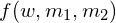表示有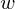位数（可以有前导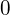）需要确定，需要让各位数字之和除以的余数为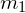，需要让这位数组成的数字除以的余数为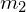需要的方案数。然后我们一步一步确定前面几位数，加起来得到答案。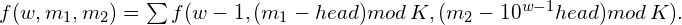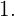记忆化搜索，那么时间复杂度为可是这么大怎么办？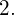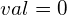时答案为不为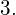可以先让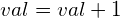，然后紧贴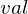求解答案，需要同时记录已经有的各位数字之和和总的数字之和。

#include<cmath>
#include<cstdio>
#include<cstring>
template<typename T>
x=0;char c;T  f=1;
do{c=getchar();if(c=='-')f=-1;else if(c==EOF)return false;}while(c>'9'||c<'0');
do{x=x*10+c-'0';c=getchar();}while(c>='0'&&c<='9');
return x*=f,true;
}
long long A,B,K;
long long tp;//tp[i]表示10 的i次方
long long Div;
long long d;

#define res1 (d[w][m1][m2])
long long f(int w,int m1,int m2){
if(w==0){
return m1==0&&m2==0;
}
if(~d[w][m1][m2])return d[w][m1][m2];
res1=0;
for(register int  i=0;i<=9;++i){
res1+=f(w-1,(m1-i%K+K)%K,(m2-(1ll*tp[w-1]*i%K)+K)%K);
//必须保证mod K之前为非负数 所以加上一个K
}
return res1;
}
long long Getws(long long val){//分割这个数，并且返回它的位数

if(!val){//其实这步可以不要。毕竟在GetAns()的入口处特判了0的情况
return Div=(Div=0)+1;
}
Div=0;
while(val){
Div[++Div]=val%10;
val/=10;
}
return  Div;
}
long long GetAns(long long val){
if(!val)return 1ll;
long long res=0;
++val;
//紧贴val来计算答案，如果不加1会漏掉val这个数
//当然也可以不加1，最后特判val这个数
long long ws=Getws(val);
if(ws*9<K)return 1ll;
long long presum=0,presum2=0;
//presum：当前累计的各位数字之和 如322的presum=3+2+2=7
//presum2：当前已确定数字组成的数：如32***的presum2=32000.
for(register int  i=ws;i>=1;--i){
for(register int  j=0;j<Div[i];++j){
if(j)presum+=1;presum%=K;
if(j)presum2+=tp[i-1];presum2%=K;
//presum,presum2每步取模更方便
res+=f(i-1,(K-presum+K)%K,(K-presum2+K)%K);
//此处有减法，必须保证它是非负数
}
//紧贴val来取值，则最高一位没有被讨论到
//例如，如果val=32565
//如果已经枚举到第二位
//那么实际上我们暂时只会先考虑30***和31***的情况
//但在后面的讨论中，应保证第二位为2
//所以还要把2的贡献累加进去
if(Div[i]){
++presum;
presum2+=tp[i-1];
}
}
return res;
}
void  work(){
long long ans=GetAns(B)-GetAns(A-1);
printf("%lld\n",ans);
}
int main(){
//freopen("testin.txt","r",stdin);
//freopen("testout.txt","w",stdout);
tp=1;
for(register int  i=1;i<=15;++i){
tp[i]=10ll*tp[i-1];
}
long long T;
while(T--){
memset(d,-1,sizeof(d));
work();
}

return 0;
}

题解 UVA1073 【Glenbow Museum】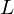个内角代表条边，根据多边形内角和公式：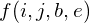表示有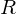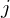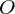，开头为表示表示），结尾为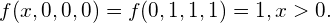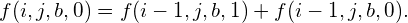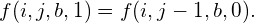（不能出现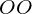#include<cstdio>
#include<cstring>
int L,kase;
long long d;
long long f(int i,int j,int b,int e){
if(~d[i][j][b][e]){
return d[i][j][b][e];
}
if(!j){
return i&&!b&&!e;
}
if(!i){
return j==1&&b==1&&e==1;
}
if(e){
return d[i][j][b][e]=f(i,j-1,b,0);
}
else{
return d[i][j][b][e]=f(i-1,j,b,0)+f(i-1,j,b,1);
}
}
int main(){
memset(d,-1,sizeof(d));
long long ans;
while(~scanf("%d",&L)&&L){
ans=0;
if(!(L&1||L<4)){
ans+=f((L+4)/2,(L-4)/2,0,0);
ans+=f((L+4)/2,(L-4)/2,1,0);
ans+=f((L+4)/2,(L-4)/2,0,1);
}
printf("Case %d: %lld\n",++kase,ans);
}
return 0;
}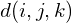表示以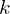开头，有，有对相邻的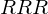算两对，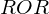对），且以结尾的方案数。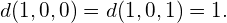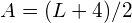#include<cstdio>
#include<cstring>
int L,kase;
long long d;
long long f(int i,int j,int k){
if(i==1){
return !j;
}
if(~d[i][j][k])return d[i][j][k];
return d[i][j][k]=f(i-1,j,k)+f(i-1,j-1,k);
}
int main(){
memset(d,-1,sizeof(d));
while(~scanf("%d",&L)&&L){
if(L&1||L<4){
printf("Case %d: 0\n",++kase);
continue;
}
printf("Case %d: %lld\n",++kase,
f((L+4)/2,3,0)+//R...R
f((L+4)/2,4,1)+//O...R
f((L+4)/2,4,0)  //R...O
);
}
return 0;
}在开头放不在开头放四个角都不放人。

(也就是说要枚举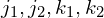)仅一个角放人。 那么又有四种情况:左上、左下、右上、右下。显然这四种是等价的，求一种之后乘以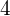即为答案。

3.有且只有两个角放人。

4.有且只有三个角放人。个点中选出个有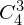种方法，除开四角有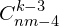种方法。

5.所有角都放人。

#include<cstdio>
#include<cstring>
// #define LOC

const long long mod=1000007;
long long C;
long long n,m,k;
long long ans;
long long T;
int kase;
void init(){
C=1;
for(register int i=1;i<=500;++i){
C[i]=1;
for(register int j=1;j<=i;++j){
C[i][j]=C[i-1][j-1]+C[i-1][j];
C[i][j]%=mod;
// printf("C[%d][%d]=%lld\n",i,j,C[i][j]);
}
}
}

void work(){
scanf("%lld%lld%lld",&n,&m,&k);
ans=0;
if(m*n>=k){
{//四个角都不放人
for(register int j1=1;j1<=n-2;++j1){
if(j1>=k)continue;
for(register int j2=1;j2<=n-2;++j2){
if(j1+j2>=k)continue;
for(register int k1=1;k1<=m-2;++k1){
if(j1+j2+k1>=k)continue;
for(register int k2=1;k2<=m-2;++k2){
if(j1+j2+k1+k2>k)continue;
ans+=C[n-2][j1]*C[n-2][j2]%mod*C[m-2][k1]%mod*C[m-2][k2]%mod*C[(m-2)*(n-2)][k-(j1+j2+k1+k2)]%mod;
ans%=mod;
}
}
}
}
ans%=mod;
}

{// 一个角上放人
for(register int j1=1;j1<=n-2&&j1<k;++j1){
for(register int k1=1;k1<=m-2;++k1){
if(j1+k1+1>k)continue;
ans+=C[n-2][j1]*C[m-2][k1]%mod*C[(m-1)*(n-1)-1][k-1-j1-k1]%mod*4%mod;
ans%=mod;
}
}
ans%=mod;
}

{//左上左下或者右上右下放置
for(register int j=1;j<=n-2&&j<=k-2;++j){
ans+=C[n-2][j]*C[m*n-n-2][k-2-j]%mod*2%mod;
ans%=mod;
}
ans%=mod;
}

{//左上右上或者左下右下放置
for(register int j=1;j<=m-2&&j<=k-2;++j){
ans+=C[m-2][j]*C[m*n-m-2][k-2-j]%mod*2%mod;
ans%=mod;
}
ans%=mod;
}

if(k>=2){//对角线上放置
ans+=2*C[m*n-4][k-2];
ans%=mod;
}

if(k>=3){//三个角都放
ans+=C*C[m*n-4][k-3]%mod;
ans%=mod;
}

if(k>=4){//四个角都放
ans+=C[m*n-4][k-4];
ans%=mod;
}
}
printf("Case %d: %lld\n",++kase,ans);
}
int main(){
scanf("%lld",&T);
init();
while(T--){
work();
}
return 0;
}

[SDOI2015]寻宝游戏 题解

题意分析

(在机房只能用Windows 7的画图工具，只能说还算能看吧)

解决方案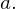假如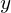在原有路径上，即加入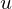之前，就被使节点连通的路径覆盖。也就是说，满足情况2或情况3。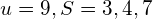能到达的 最近的 子树中有节点在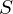中的 点。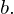假如不在原有路径上，那么以的子树外一定没有节点在中。（否则满足情况3，原有路径一定经过可知，无论选择哪个点，都有一段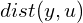，而这段距离是已固定的。（当前只有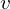不确定）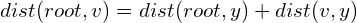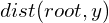是恒定的。

代码实现

1.节点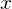的子树中有多少节点属于2.离节点最近的 子树中有节点属于的 点；

3.树上任意两点间的距离；

4.已经被路径覆盖的点中，距离根最近的点。的子树中的点就是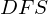序在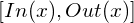中间的点。

（又巧了，倍增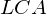不正好维护了每个节点的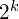倍祖先么？）

#include<cmath>
#include<queue>
#include<cstdio>
#include<cstring>

//#define LOC
#define nowy (L[i].to)
using std::queue;
using std::priority_queue;
template <typename T>
x=0;char c; T f=1;
do{c=getchar();if(c=='-')f=-1;}while(c>'9'||c<'0');
do{x=x*10+c-'0';c=getchar();}while(c>='0'&&c<='9');x*=f;
}

const int N=1e5 + 10;

int n,m,t;
int h[N],tot=1;
int f[N];
int d[N];
long long len[N];
int bg[N],ed[N];
int Inq[N];
int DFN;
int TotIn;
int Diff[N];//Diff是FT的一个拷贝 便于直接确认一个点是否在S中
int x,y,z;
int Num_SonIn,Cls_F;
long long ans=0;

struct line{
int to,nxt,w;
}L[2*N];

struct OneNode{
int num;
bool operator <(const OneNode b)const {
if(len[num]==len[b.num])return d[num]>d[b.num];
return len[num]>len[b.num];
}
OneNode(int x):num(x){}
};//通过便于维护小根堆
struct FenwickTree{
int v[N];
inline void clear(){
memset(v,0,sizeof(v));
}
for(;x<=n;x+=x&-x){
v[x]+=y;
}
}
int res=0;
while(x>0){
res+=v[x];
x-=x&-x;
}
return res;
}
FenwickTree(){clear();}
}FT;
priority_queue<OneNode> OQ;

inline void add(int x,int y,int z){
L[++tot].to=y;L[tot].nxt=h[x];h[x]=tot;L[tot].w=z;
L[++tot].to=x;L[tot].nxt=h[y];h[y]=tot;L[tot].w=z;
}

void GetLCA_DFS(int x){
//DFS 同时求出祖先数组、距离根节点的距离、DFS序
bg[x]=++DFN;
for(int i=h[x];i;i=L[i].nxt){
if(d[nowy])continue;
d[nowy]=d[x]+1;
f[nowy]=x;
len[nowy]=len[x]+L[i].w;
for(int j=1;j<=t;++j){
f[nowy][j]=f[f[nowy][j-1]][j-1];
}
GetLCA_DFS(nowy);
}
ed[x]=DFN;
}
inline int LCA(int x,int y){
if(d[x]>d[y]){
x^=y;y^=x;x^=y;
}
//d[x]<=d[y]
for(register int i=t;i>=0;--i){
if(d[f[y][i]]>=d[x]){
y=f[y][i];
}
}
if(x==y)return x;
for(register int i=t;i>=0;--i){
if(f[y][i]!=f[x][i]){
y=f[y][i];
x=f[x][i];
}
}
return f[x];
}
inline long long LEN(int x,int y){
return 1ll*len[x]+len[y]-2*len[LCA(x,y)];
}
inline int GetSonIn(int x){//including x
//判断x的子树中有多少节点属于S
return Num;
}
inline bool TwoSon(int x,int v){
//是否满足情况2
int SonV;
for(register int i=h[x];i;i=L[i].nxt){
if(d[nowy]<=d[x])continue;
SonV=GetSonIn(nowy);

if(SonV){
if(SonV==v)return false;
return true;
}
}
return false;
}
inline bool check(int x){
//是否满足情况2或3 这两种情况可以一同处理
if(!x)return true;
int Num_SonIn;
return (Num_SonIn=GetSonIn(x))&&(Num_SonIn<TotIn||TwoSon(x,Num_SonIn));
}
inline int GetCls_F(int x){
//倍增求离自己最近的 子树里有节点属于S 的节点
if(GetSonIn(x))return x;
int Num;
for(register int i=t;i>=0;--i){
if(!(Num=GetSonIn(f[x][i]))){
x=f[x][i];
}
}
return f[x];
}
inline void Change(long long t){
//通过t变量实现增加删除在同一个函数处理 减少码量
if(TotIn!=0){
if(!check(x)){
Cls_F=GetCls_F(x);
if(check(Cls_F)){
if(t&&!Inq[Cls_F]){OQ.push(OneNode(Cls_F));Inq[Cls_F]=1;}
ans+=LEN(x,Cls_F)*(-1+2*t);
}
else {
while(!OQ.empty()){
z=OQ.top().num;
if(check(z)||Diff[z])break;
OQ.pop();
Inq[z]=0;
}
if(t&&!Inq[Cls_F]){OQ.push(OneNode(Cls_F));Inq[Cls_F]=1;}
ans+=LEN(x,z)*(-1+2*t);
}
}
}
}
int main(){
t=log(n)/log(2)+1;
for(register int i=1;i<n;++i){
}
d=1;
GetLCA_DFS(1);
for(register int i=1;i<=m;++i){
if(!Diff[x]){
Change(1);
Diff[x]+=1;
if(!Inq[x]){Inq[x]=1;OQ.push(OneNode(x));}
++TotIn;
}
else{
--TotIn;
Diff[x]-=1;

Change(0);
}
printf("%lld\n",ans*2);
}
return 0;
}

Uae牛逼！

/*******/